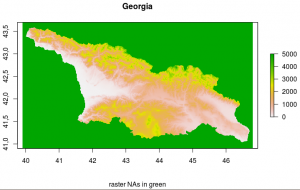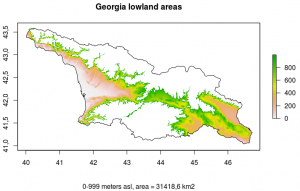# Raster Basics 3 – Area Calculations

Spatial Analyses include calculations of area sizes in study regions. In R such analyses can be based on either shapefiles or raster objects.

A straightforward approach is calculating the area from a shapefile using the „areaPolygon“function of R’s „geosphere package“

#get shapefile of Georgia’s borders
#calculate area [m2] of the polygon
sqm<-areaPolygon(geo_border)
#convert sqm to km2
sqkm<-sqm/1000000
#print area of Georgia according to shapefile, rounded to one digit
print(paste(„Area of Georgia (shapefile):“,round(sqkm, digits=1),“km2″))

In this case, the output would be: „Area of Georgia (shapefile): 69,890.7 km2“. This is pretty close to the 69,700 km2 given in Wikipedia.Raster of SRTM data of Georgia. The raster’s NA values are colored green. These need to be deleted from the raster in order to calculate the country area.

Alternatively, the area can be calculated based on the raster object. The „area“ function of R’s „raster package“ results in a vector of the cell sizes in the raster object (in km2), which differ from north to south. In order to calculate the entire area’s size can be obtained by adding up all cell sizes or multiplying the median cell size by the number of cells (which I did here).

In these calculations all cells with ‚NA‘ values need to be eliminated. These cells represent the „unused“ cells outside the country limits (see map with green NA values) and should not be used in the calculation of the country’s area.

##getting SRTM data of Georgia
#get sizes of all cells in raster [km2]
cell_size<-area(geo_raster, na.rm=TRUE, weights=FALSE)
#delete NAs from vector of all raster cells
##NAs lie outside of the rastered region, can thus be omitted
cell_size<-cell_size[!is.na(cell_size)]
#compute area [km2] of all cells in geo_raster
raster_area<-length(cell_size)*median(cell_size)
#print area of Georgia according to raster object
print(paste(„Area of Georgia (raster):“,round(raster_area, digits=1),“km2″))Map of all regions in Georgia between 0 and 999 m above sea level (i.e. lowland regions).

The output would be: „Area of Georgia (raster): 70010.1 km2“. This differs by only 0.18 percent from the area calculated from the shapefile.

By subsetting the raster object and selectively converting values to NAs it is possible to plot only a certain altitudinal zone and calculate its area:

##lowland zone 0-999 m
geo_raster1000 <- geo_raster
geo_raster1000[geo_raster1000 <=-1] <- NA
geo_raster1000[geo_raster1000 >999] <- NA
#calculate area of regions under 0 m asl
#get sizes of all cells under 0 m
cell_size<-area(geo_raster1000, na.rm=TRUE, weights=FALSE)
#delete NAs from vector of all raster cells
##NAs lie outside of the rastered region, can thus be omitted
cell_size<-cell_size[!is.na(cell_size)]
#compute area [km2] of all cells in geo_raster1000
lowland_area<-length(cell_size)*median(cell_size)
#print area of Georgia according to raster object
print(paste(„Area of lowland regions (0-999 m):“,round(lowland_area, digits=1),“km2″))
#plot lowland zone
X11(width=8, height=5)
plot(geo_raster1000,width=15, height=10,main=“Georgia lowland areas“,sub=paste(„0-999 meters asl, area =“,round(lowland_area, digits=1),“km2″))

All these calculations can be reproduced using the R code in area_script.txt. Using it, a rough estimation of the altitudinal/vegetation zones in Georgia can be made (see table).

zone Elevation Range
[m above Sea level]
Area [square km] Relative Proportion in Percent
(total 70010.1 square km)
nival 4000+ 54.2 0.1
alpine 2400-4000 6990.7 10.0
montane 1000-2399 31436.5 44.9
lowland 1-999 31418.6 44.9
below zero <0 49.6 0.1

Diese Website verwendet Akismet, um Spam zu reduzieren. Erfahre mehr darüber, wie deine Kommentardaten verarbeitet werden.